# Chapter 17 Stacks and Queues Java Programming Program

• Slides: 87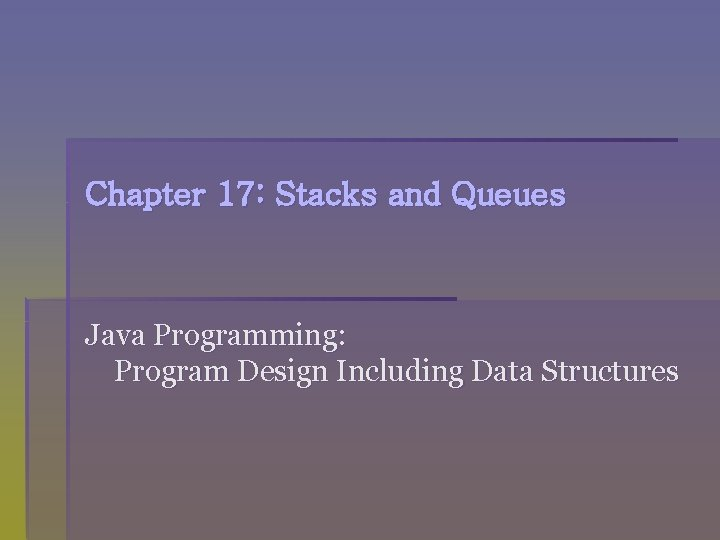Chapter 17: Stacks and Queues Java Programming: Program Design Including Data StructuresChapter Objectives s s Learn about stacks Examine various stack operations Learn how to implement a stack as an array Learn how to implement a stack as a linked list Discover stack applications Java Programming: Program Design Including Data Structures 2Chapter Objectives (continued) s s s Learn how to use a stack to remove recursion Learn about queues Examine various queue operations Learn how to implement a queue as an array Discover queue applications Java Programming: Program Design Including Data Structures 3Stacks s Lists of homogeneous elements s Addition and deletion of elements occur only at one end, called the top of the stack s Computers use stacks to implement method calls s Stacks are also used to convert recursive algorithms into nonrecursive algorithms s Especially recursive algorithms that are not tail recursive Java Programming: Program Design Including Data Structures 4Stacks (continued) Figure 17 -1 Various types of stacks Java Programming: Program Design Including Data Structures 5Stacks (continued) s Stacks are also called Last Input First Output (LIFO) data structures s Operations performed on stacks s Push: adds an element to the stack s Pop: removes an element from the stack s Peek: looks at the top element of the stack Java Programming: Program Design Including Data Structures 6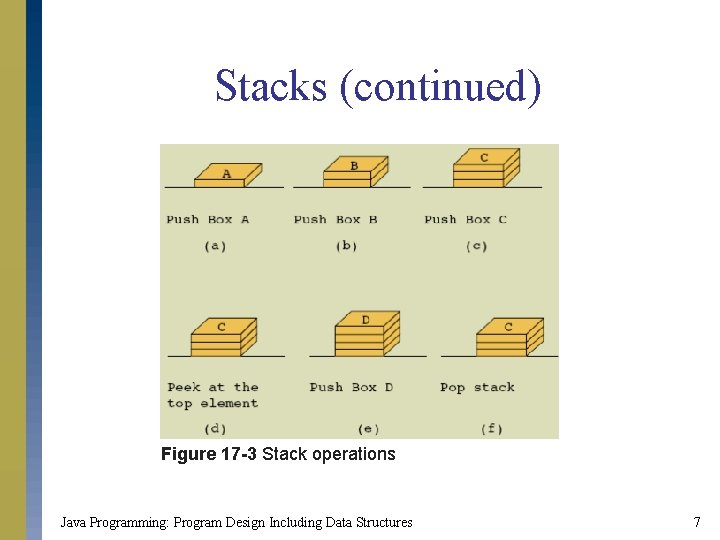Stacks (continued) Figure 17 -3 Stack operations Java Programming: Program Design Including Data Structures 7Stacks (continued) Figure 17 -4 UML diagram of the interface Stack. ADT public class Stack. ADT<T> { … } Java Programming: Program Design Including Data Structures 8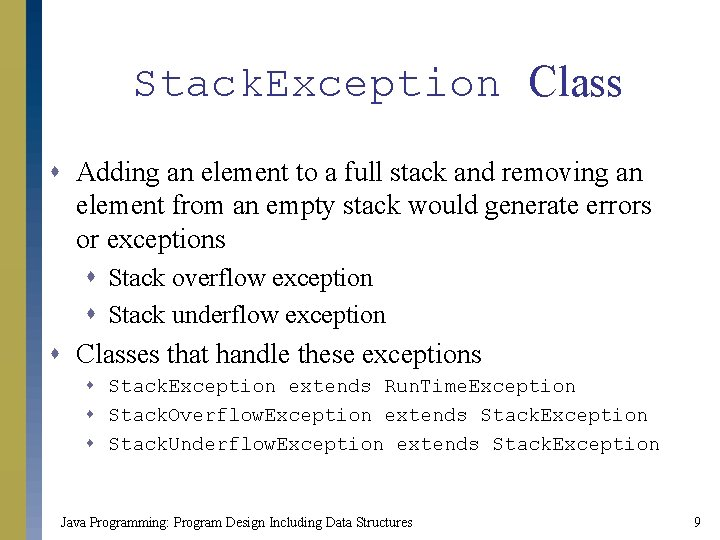Stack. Exception Class s Adding an element to a full stack and removing an element from an empty stack would generate errors or exceptions s Stack overflow exception s Stack underflow exception s Classes that handle these exceptions s Stack. Exception extends Run. Time. Exception s Stack. Overflow. Exception extends Stack. Exception s Stack. Underflow. Exception extends Stack. Exception Java Programming: Program Design Including Data Structures 9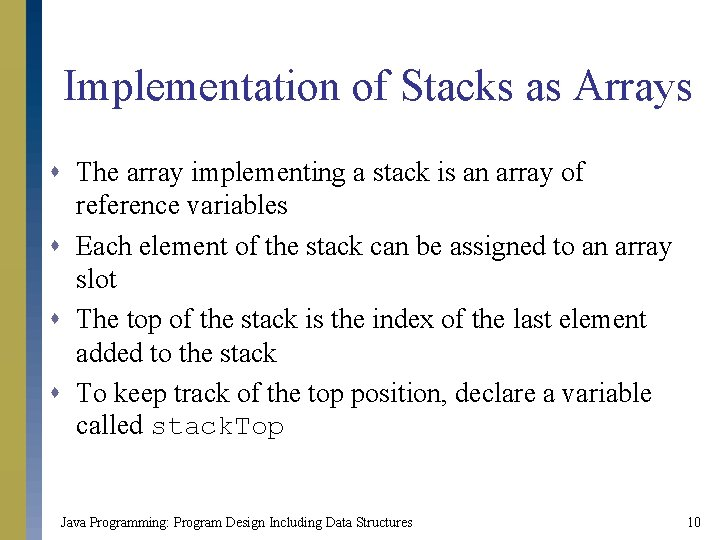Implementation of Stacks as Arrays s The array implementing a stack is an array of reference variables s Each element of the stack can be assigned to an array slot s The top of the stack is the index of the last element added to the stack s To keep track of the top position, declare a variable called stack. Top Java Programming: Program Design Including Data Structures 10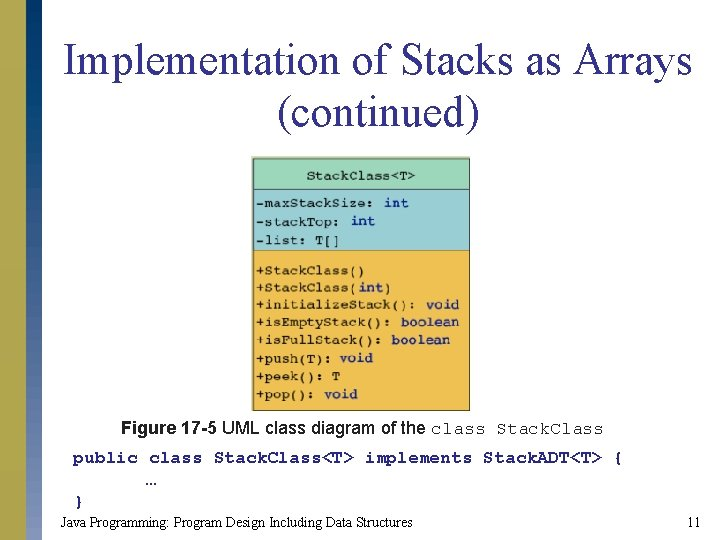Implementation of Stacks as Arrays (continued) Figure 17 -5 UML class diagram of the class Stack. Class public class Stack. Class<T> implements Stack. ADT<T> { … } Java Programming: Program Design Including Data Structures 11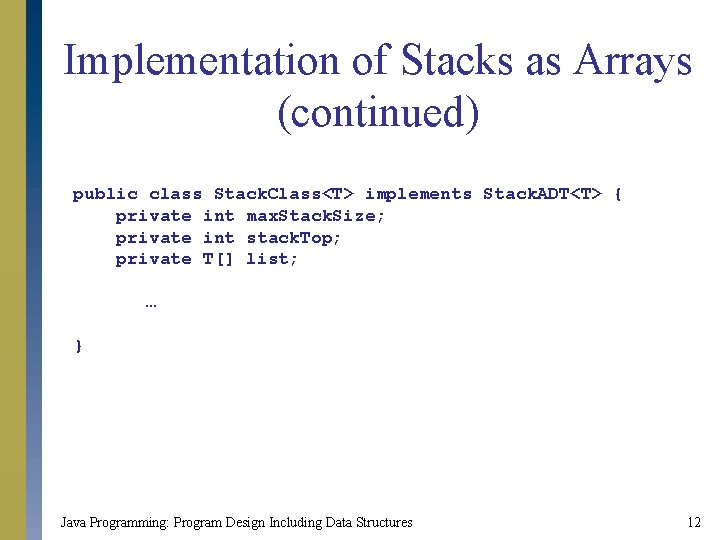Implementation of Stacks as Arrays (continued) public class Stack. Class<T> implements Stack. ADT<T> { private int max. Stack. Size; private int stack. Top; private T[] list; … } Java Programming: Program Design Including Data Structures 12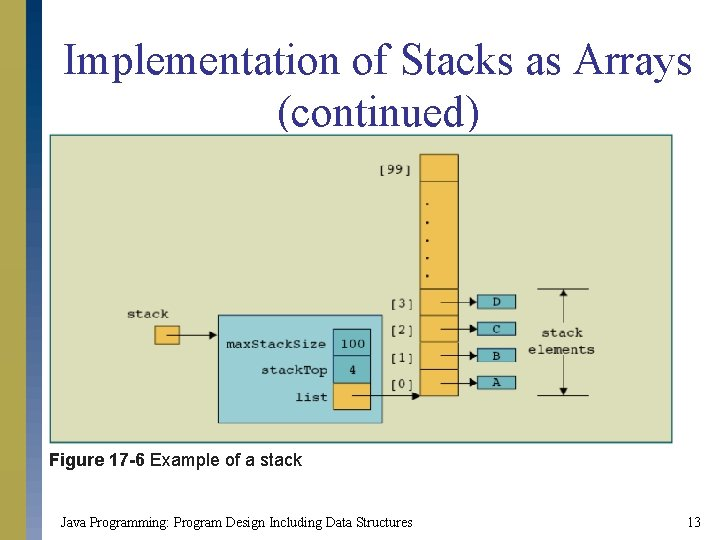Implementation of Stacks as Arrays (continued) Figure 17 -6 Example of a stack Java Programming: Program Design Including Data Structures 13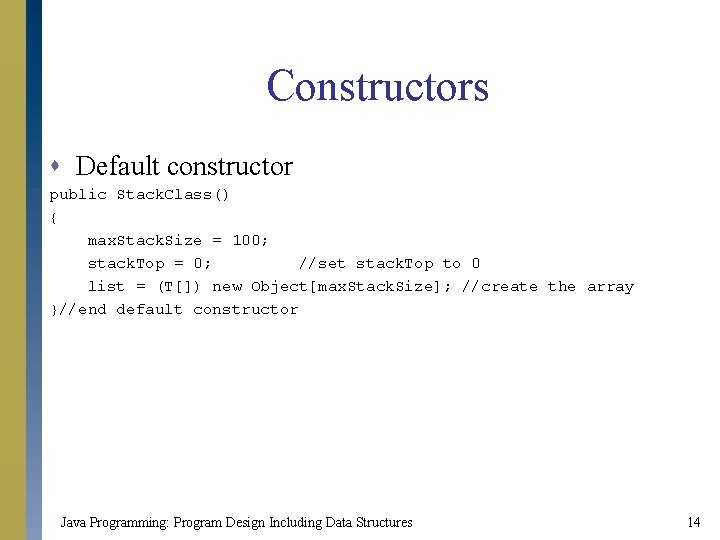Constructors s Default constructor public Stack. Class() { max. Stack. Size = 100; stack. Top = 0; //set stack. Top to 0 list = (T[]) new Object[max. Stack. Size]; //create the array }//end default constructor Java Programming: Program Design Including Data Structures 14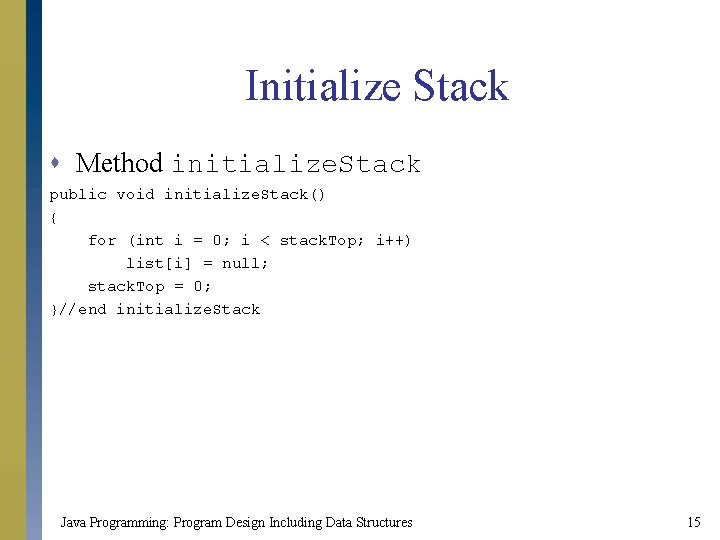Initialize Stack s Method initialize. Stack public void initialize. Stack() { for (int i = 0; i < stack. Top; i++) list[i] = null; stack. Top = 0; }//end initialize. Stack Java Programming: Program Design Including Data Structures 15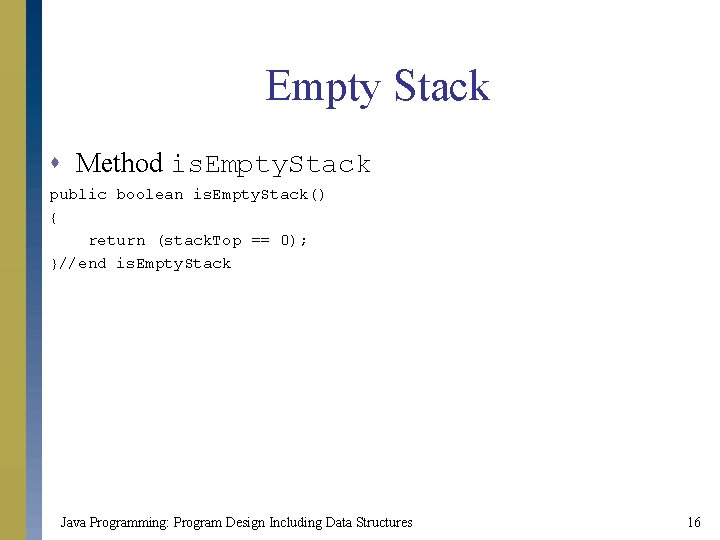Empty Stack s Method is. Empty. Stack public boolean is. Empty. Stack() { return (stack. Top == 0); }//end is. Empty. Stack Java Programming: Program Design Including Data Structures 16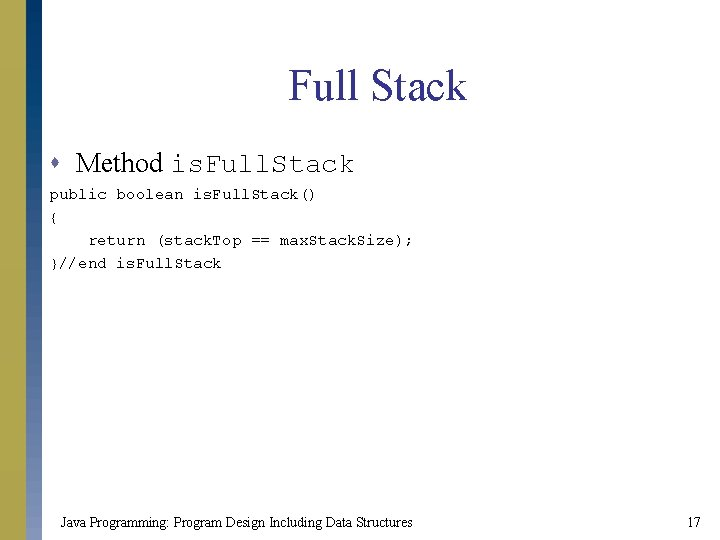Full Stack s Method is. Full. Stack public boolean is. Full. Stack() { return (stack. Top == max. Stack. Size); }//end is. Full. Stack Java Programming: Program Design Including Data Structures 17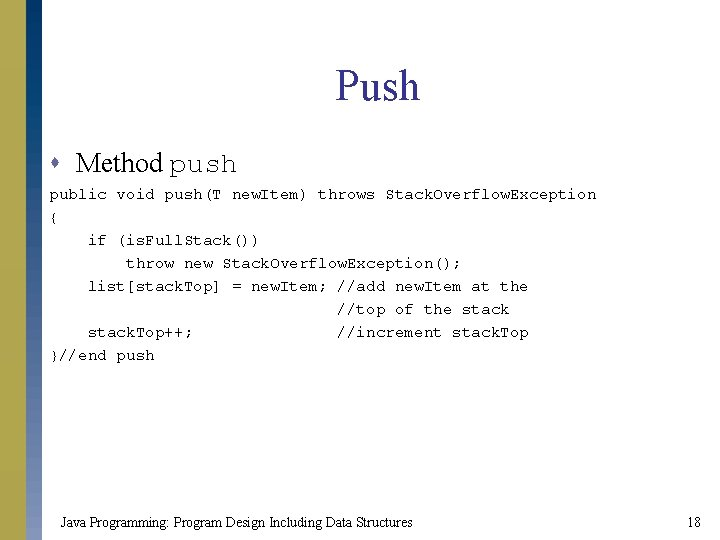Push s Method push public void push(T new. Item) throws Stack. Overflow. Exception { if (is. Full. Stack()) throw new Stack. Overflow. Exception(); list[stack. Top] = new. Item; //add new. Item at the //top of the stack. Top++; //increment stack. Top }//end push Java Programming: Program Design Including Data Structures 18Peek s Method peek public T peek() throws Stack. Underflow. Exception { if (is. Empty. Stack()) throw new Stack. Underflow. Exception(); return (T) list[stack. Top - 1]; }//end peek Java Programming: Program Design Including Data Structures 19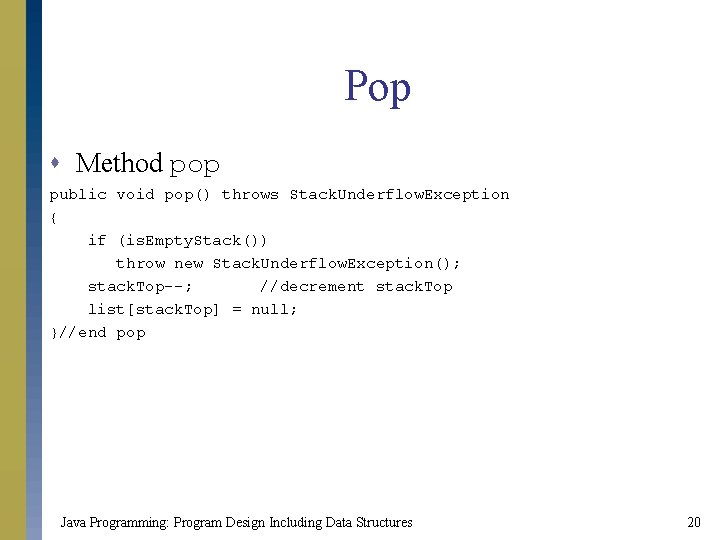Pop s Method pop public void pop() throws Stack. Underflow. Exception { if (is. Empty. Stack()) throw new Stack. Underflow. Exception(); stack. Top--; //decrement stack. Top list[stack. Top] = null; }//end pop Java Programming: Program Design Including Data Structures 20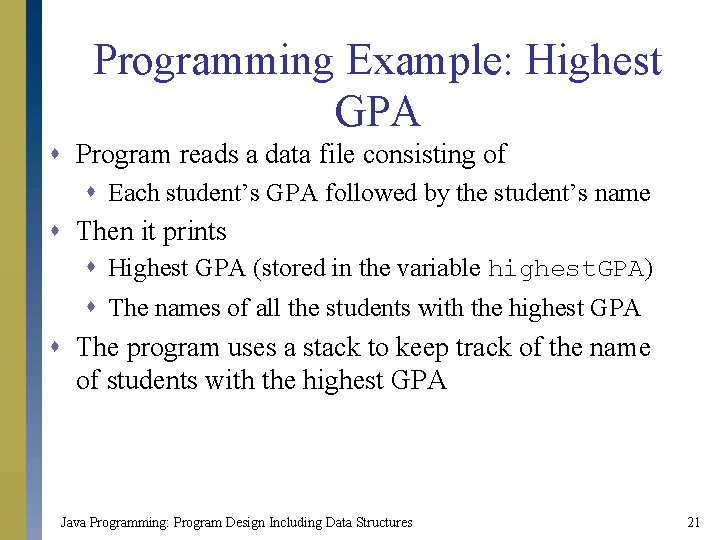Programming Example: Highest GPA s Program reads a data file consisting of s Each student’s GPA followed by the student’s name s Then it prints s Highest GPA (stored in the variable highest. GPA) s The names of all the students with the highest GPA s The program uses a stack to keep track of the name of students with the highest GPA Java Programming: Program Design Including Data Structures 21Linked Implementation of Stacks s Arrays have fixed sizes s Only a fixed number of elements can be pushed onto the stack s Dynamically allocate memory using reference variables s Implement a stack dynamically s Similar to the array representation, stack. Top is used to locate the top element s stack. Top is now a reference variable Java Programming: Program Design Including Data Structures 22Linked Implementation of Stacks (continued) Figure 17 -13 Nonempty linked stack public class Linked. Stack. Class<T> implements Stack. ADT<T> { private Stack. Node<T> stack. Top; … } Java Programming: Program Design Including Data Structures 23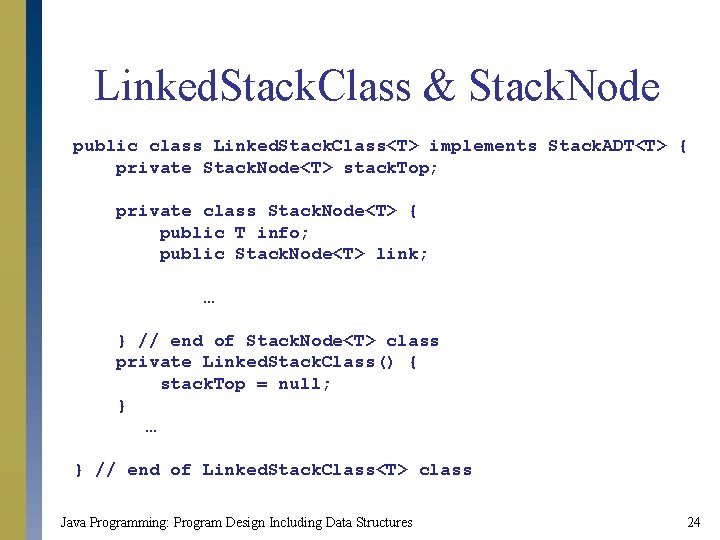Linked. Stack. Class & Stack. Node public class Linked. Stack. Class<T> implements Stack. ADT<T> { private Stack. Node<T> stack. Top; private class Stack. Node<T> { public T info; public Stack. Node<T> link; … } // end of Stack. Node<T> class private Linked. Stack. Class() { stack. Top = null; } … } // end of Linked. Stack. Class<T> class Java Programming: Program Design Including Data Structures 24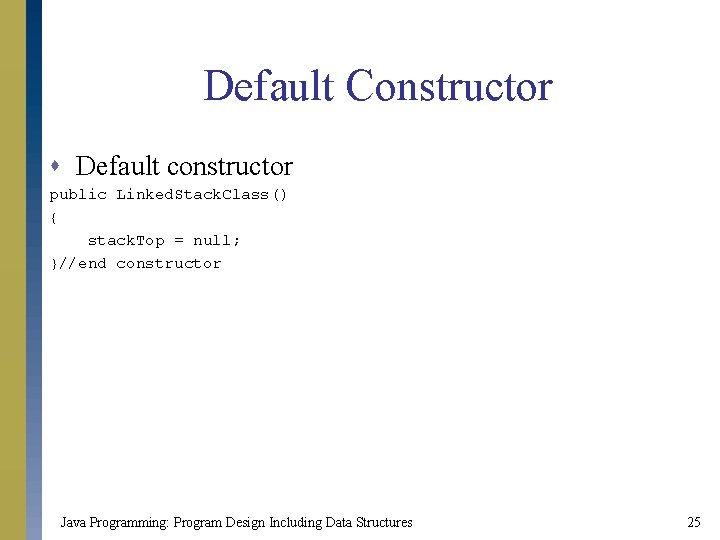Default Constructor s Default constructor public Linked. Stack. Class() { stack. Top = null; }//end constructor Java Programming: Program Design Including Data Structures 25Initialize Stack s Method initialize. Stack public void initialize. Stack() { stack. Top = null; }//end initialize. Stack Java Programming: Program Design Including Data Structures 26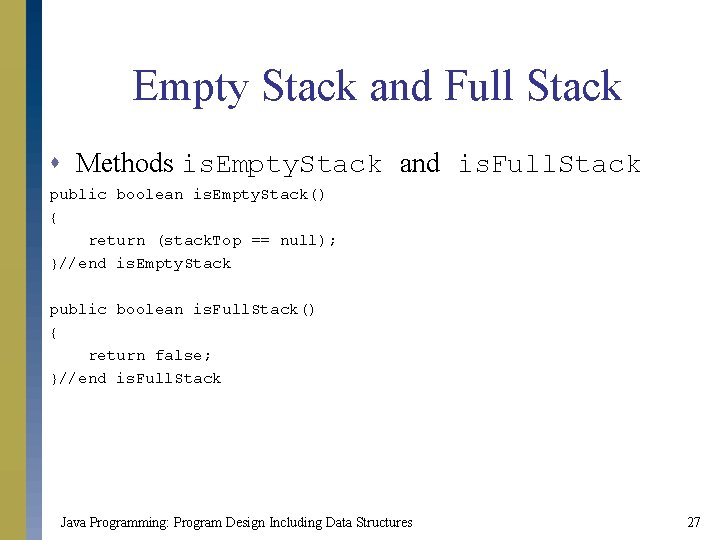Empty Stack and Full Stack s Methods is. Empty. Stack and is. Full. Stack public boolean is. Empty. Stack() { return (stack. Top == null); }//end is. Empty. Stack public boolean is. Full. Stack() { return false; }//end is. Full. Stack Java Programming: Program Design Including Data Structures 27Push s Method push public void push(T new. Element) { Stack. Node<T> new. Node; //reference variable to create //the new node new. Node = new Stack. Node<T>(new. Element, stack. Top); //create //new. Node and insert //before stack. Top = new. Node; //set stack. Top to point to //the top element } //end push Java Programming: Program Design Including Data Structures 28Peek s Method peek public T peek() throws Stack. Underflow. Exception { if (stack. Top == null) throw new Stack. Underflow. Exception(); return stack. Top. info; }//end top Java Programming: Program Design Including Data Structures 29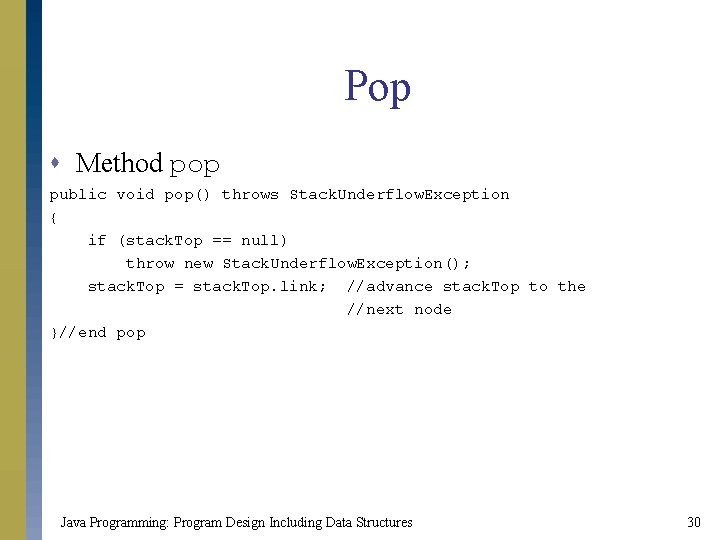Pop s Method pop public void pop() throws Stack. Underflow. Exception { if (stack. Top == null) throw new Stack. Underflow. Exception(); stack. Top = stack. Top. link; //advance stack. Top to the //next node }//end pop Java Programming: Program Design Including Data Structures 30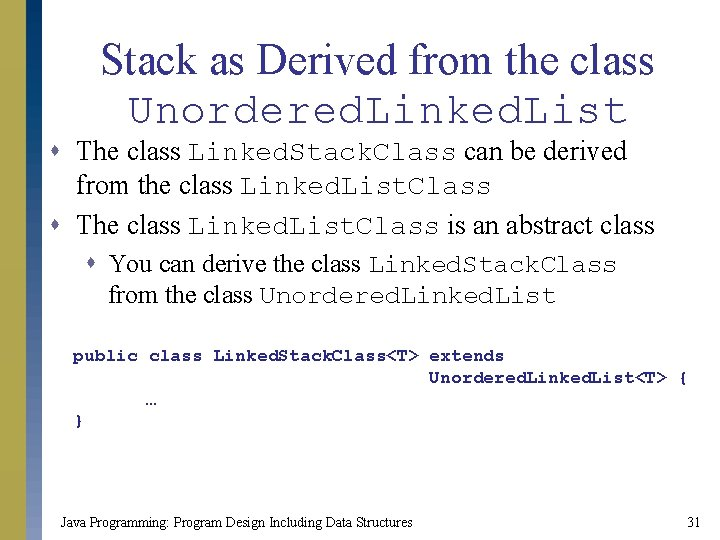Stack as Derived from the class Unordered. Linked. List s The class Linked. Stack. Class can be derived from the class Linked. List. Class s The class Linked. List. Class is an abstract class s You can derive the class Linked. Stack. Class from the class Unordered. Linked. List public class Linked. Stack. Class<T> extends Unordered. Linked. List<T> { … } Java Programming: Program Design Including Data Structures 31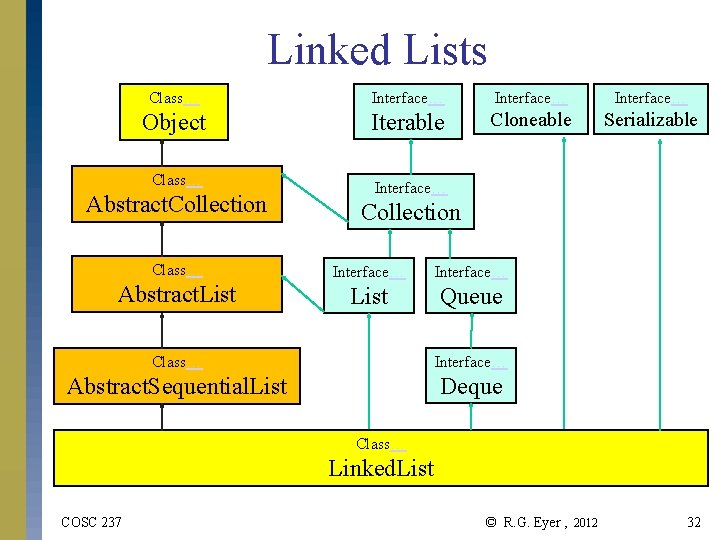Linked Lists Class… Interface… Object Iterable Cloneable Serializable Class… Interface… Abstract. Collection Class… Interface… Abstract. List Queue Class… Interface… Abstract. Sequential. List Deque Class… Linked. List COSC 237 © R. G. Eyer , 2012 32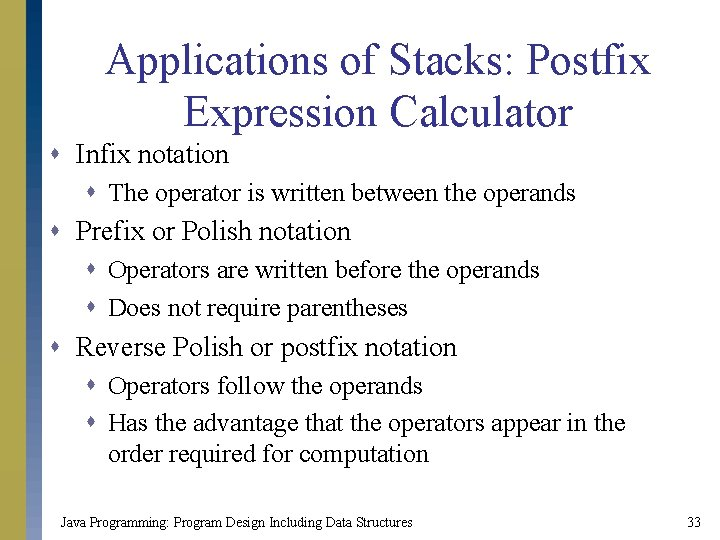Applications of Stacks: Postfix Expression Calculator s Infix notation s The operator is written between the operands s Prefix or Polish notation s Operators are written before the operands s Does not require parentheses s Reverse Polish or postfix notation s Operators follow the operands s Has the advantage that the operators appear in the order required for computation Java Programming: Program Design Including Data Structures 33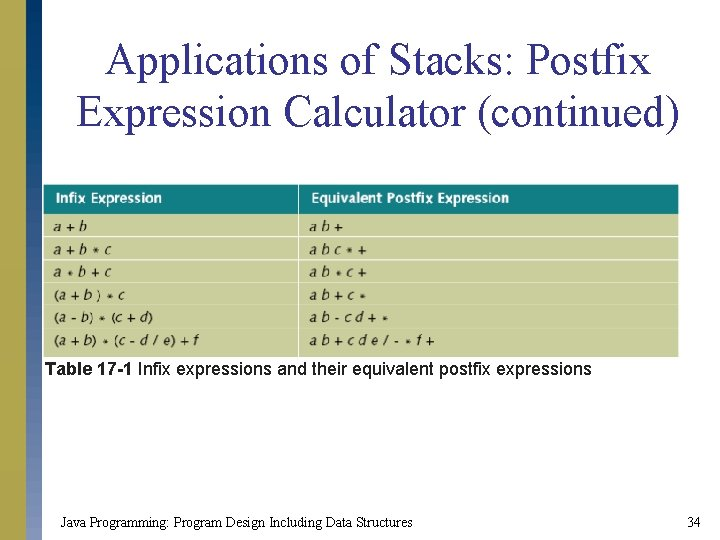Applications of Stacks: Postfix Expression Calculator (continued) Table 17 -1 Infix expressions and their equivalent postfix expressions Java Programming: Program Design Including Data Structures 34Applications of Stacks: Postfix Expression Calculator (continued) s Algorithm to evaluate postfix expressions using a stack s Scan the expression from left to right s Operands are pushed onto the stack s When an operator is found, back up to get the required number of operands s Perform the operation s Continue processing the expression Java Programming: Program Design Including Data Structures 35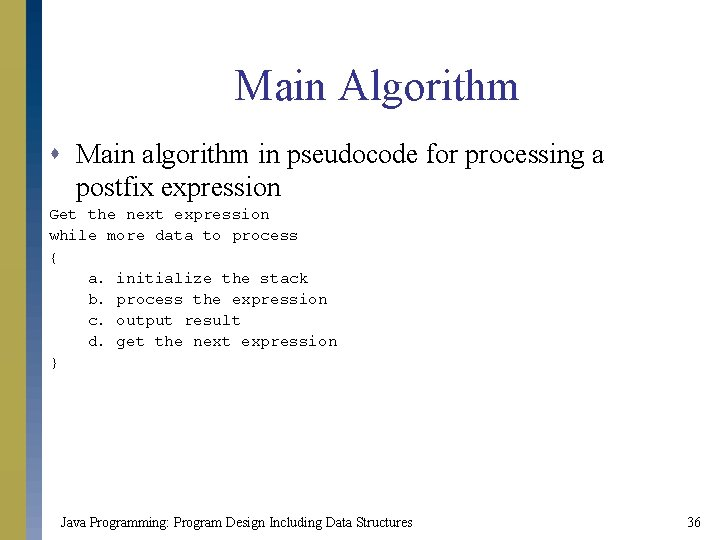Main Algorithm s Main algorithm in pseudocode for processing a postfix expression Get the next expression while more data to process { a. initialize the stack b. process the expression c. output result d. get the next expression } Java Programming: Program Design Including Data Structures 36Method evaluate. Expression s General algorithm for evaluate. Expression get the next token while (token != '=') { if (token is a number) { output number push number onto stack } else { token is an operation call method evaluate. Opr to evaluate the operation } if (no error in the expression) get next token else discard the expression } Java Programming: Program Design Including Data Structures 37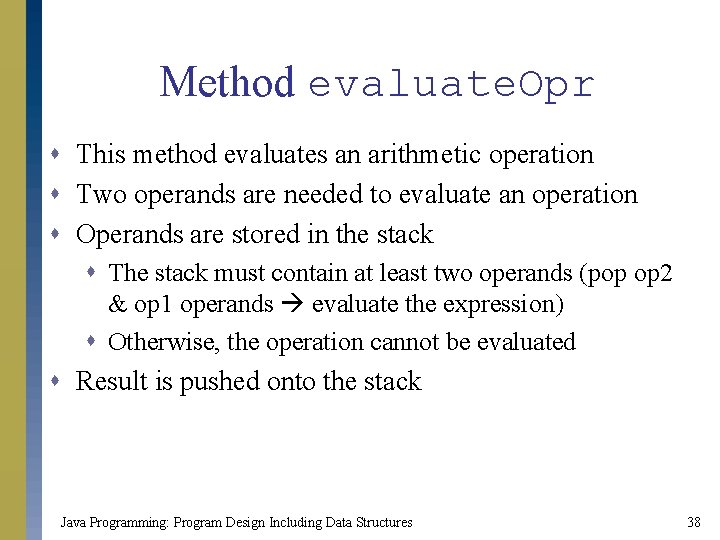Method evaluate. Opr s This method evaluates an arithmetic operation s Two operands are needed to evaluate an operation s Operands are stored in the stack s The stack must contain at least two operands (pop op 2 & op 1 operands evaluate the expression) s Otherwise, the operation cannot be evaluated s Result is pushed onto the stack Java Programming: Program Design Including Data Structures 38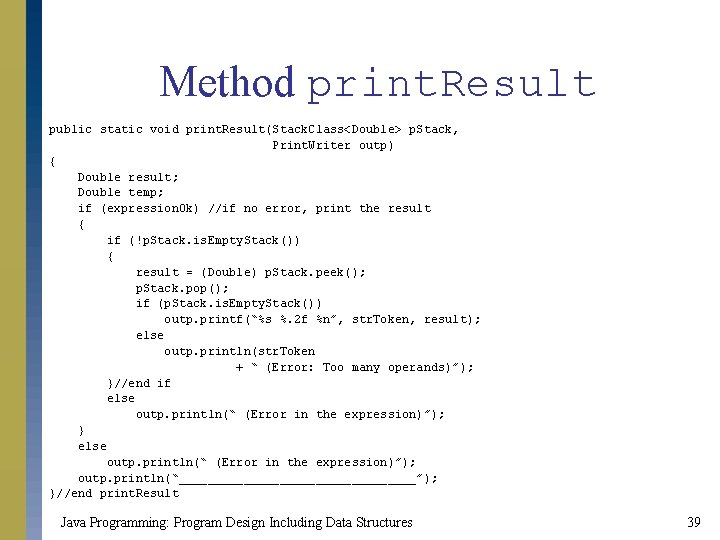Method print. Result public static void print. Result(Stack. Class<Double> p. Stack, Print. Writer outp) { Double result; Double temp; if (expression. Ok) //if no error, print the result { if (!p. Stack. is. Empty. Stack()) { result = (Double) p. Stack. peek(); p. Stack. pop(); if (p. Stack. is. Empty. Stack()) outp. printf(“%s %. 2 f %n”, str. Token, result); else outp. println(str. Token + “ (Error: Too many operands)”); }//end if else outp. println(“ (Error in the expression)”); } else outp. println(“ (Error in the expression)”); outp. println(“_________________”); }//end print. Result Java Programming: Program Design Including Data Structures 39Postfix Expression Calculator: Graphical User Interface (GUI) Figure 17 -28 GUI for the postfix calculator Java Programming: Program Design Including Data Structures 40Postfix Expression Calculator: Graphical User Interface (GUI) (continued) s Methods evaluate. Expression, evaluate. Opr, and print. Result need minor modifications s The data are no longer coming from a file s The output is no longer stored in a file s To simplify the accessing of the stack s Declare it as an instance variable of the class containing the GUI program Java Programming: Program Design Including Data Structures 41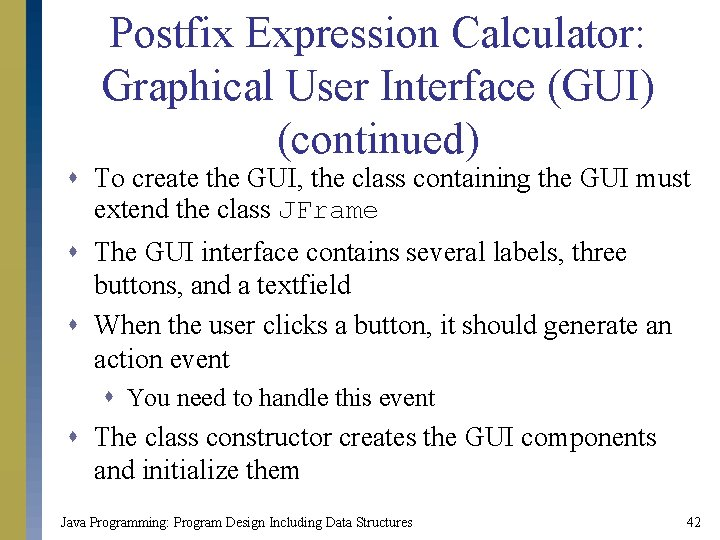Postfix Expression Calculator: Graphical User Interface (GUI) (continued) s To create the GUI, the class containing the GUI must extend the class JFrame s The GUI interface contains several labels, three buttons, and a textfield s When the user clicks a button, it should generate an action event s You need to handle this event s The class constructor creates the GUI components and initialize them Java Programming: Program Design Including Data Structures 42Postfix Expression Calculator: Graphical User Interface (GUI) (continued) Figure 17 -29 Sample run of Postfix. Calculator program Java Programming: Program Design Including Data Structures 43Removing Recursion: Nonrecursive Algorithm to Print a Linked List Backward s Naïve approach s Get the last node of the list and print it s Traverse the link starting at the first node s Repeat this process for every node s Traverse the link starting at the first node until you reach the desired node s Very inefficient Java Programming: Program Design Including Data Structures 44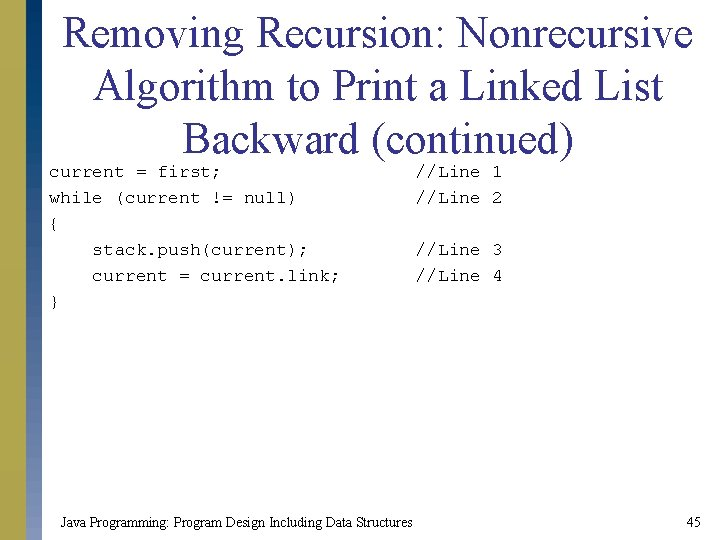Removing Recursion: Nonrecursive Algorithm to Print a Linked List Backward (continued) current = first; while (current != null) { stack. push(current); current = current. link; } Java Programming: Program Design Including Data Structures //Line 1 //Line 2 //Line 3 //Line 4 45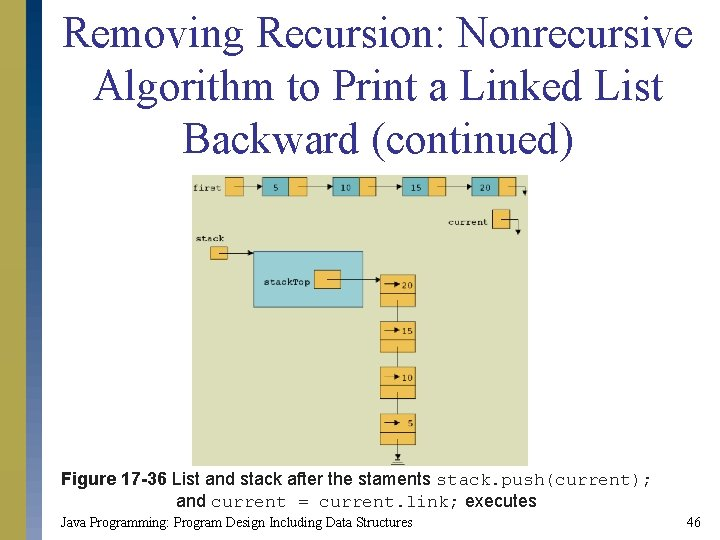Removing Recursion: Nonrecursive Algorithm to Print a Linked List Backward (continued) Figure 17 -36 List and stack after the staments stack. push(current); and current = current. link; executes Java Programming: Program Design Including Data Structures 46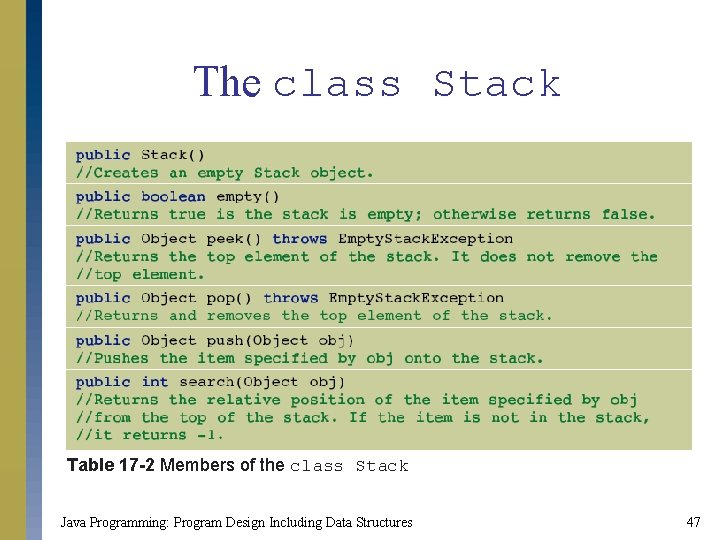The class Stack Table 17 -2 Members of the class Stack Java Programming: Program Design Including Data Structures 47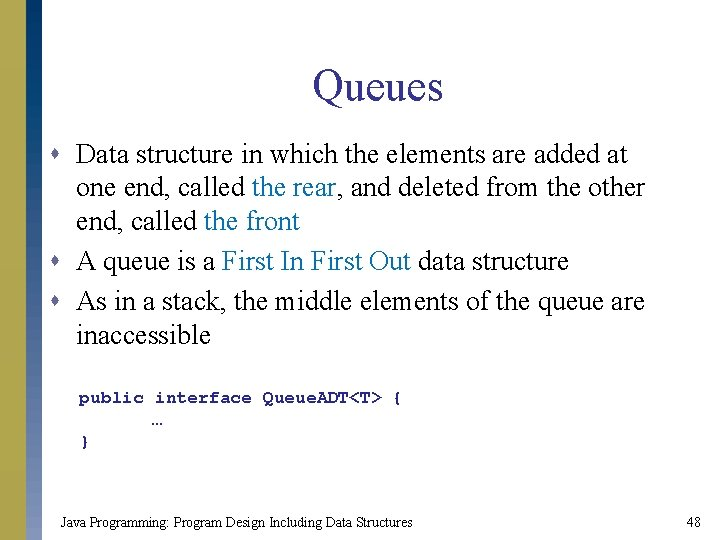Queues s Data structure in which the elements are added at one end, called the rear, and deleted from the other end, called the front s A queue is a First In First Out data structure s As in a stack, the middle elements of the queue are inaccessible public interface Queue. ADT<T> { … } Java Programming: Program Design Including Data Structures 48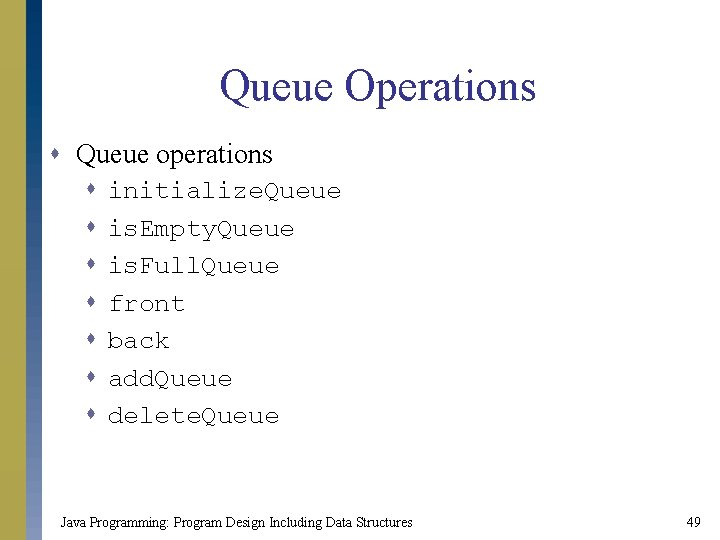Queue Operations s Queue operations s s s initialize. Queue is. Empty. Queue is. Full. Queue front back add. Queue delete. Queue Java Programming: Program Design Including Data Structures 49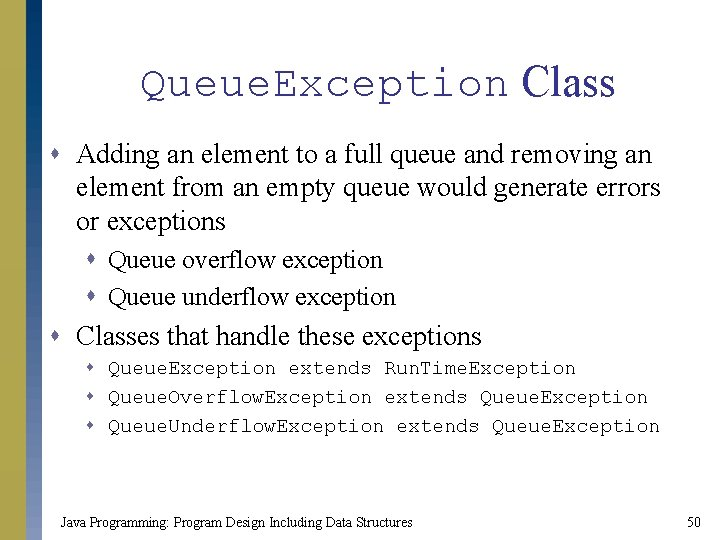Queue. Exception Class s Adding an element to a full queue and removing an element from an empty queue would generate errors or exceptions s Queue overflow exception s Queue underflow exception s Classes that handle these exceptions s Queue. Exception extends Run. Time. Exception s Queue. Overflow. Exception extends Queue. Exception s Queue. Underflow. Exception extends Queue. Exception Java Programming: Program Design Including Data Structures 50Implementation of Queues as Arrays s Instance variables s s An array to store the queue elements queue. Front: keeps track of the first element queue. Rear: keeps track of the last element max. Queue. Size: specifies the maximum size of the queues Java Programming: Program Design Including Data Structures 51Implementation of Queues as Arrays (continued) Figure 17 -42 Queue after two more add. Queue operations public class Queue. Class<T> implements Queue. ADT<T> { … } Java Programming: Program Design Including Data Structures 52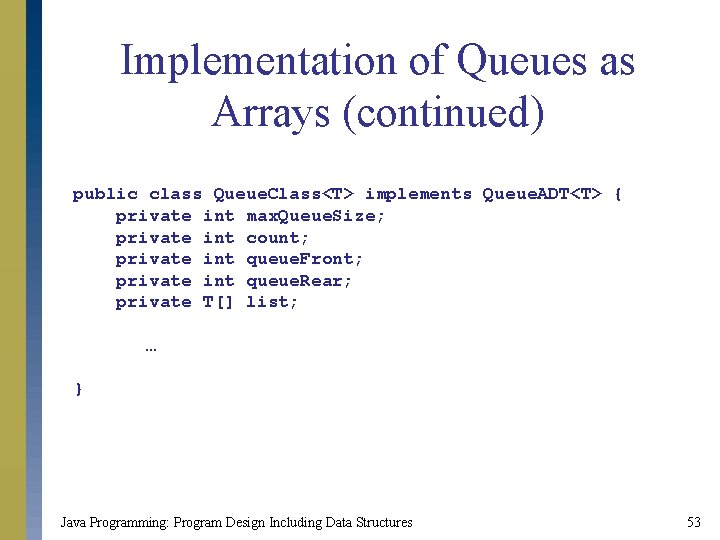Implementation of Queues as Arrays (continued) public class Queue. Class<T> implements Queue. ADT<T> { private int max. Queue. Size; private int count; private int queue. Front; private int queue. Rear; private T[] list; … } Java Programming: Program Design Including Data Structures 53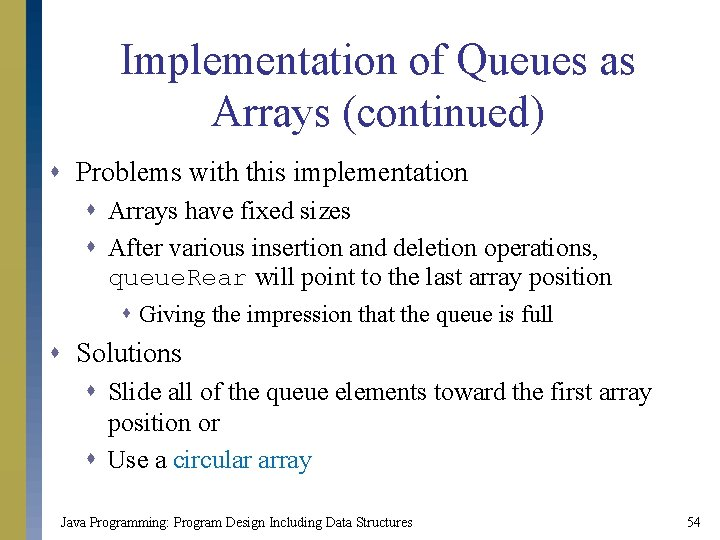Implementation of Queues as Arrays (continued) s Problems with this implementation s Arrays have fixed sizes s After various insertion and deletion operations, queue. Rear will point to the last array position s Giving the impression that the queue is full s Solutions s Slide all of the queue elements toward the first array position or s Use a circular array Java Programming: Program Design Including Data Structures 54Implementation of Queues as Arrays (continued) Figure 17 -45 Circular queue Java Programming: Program Design Including Data Structures 55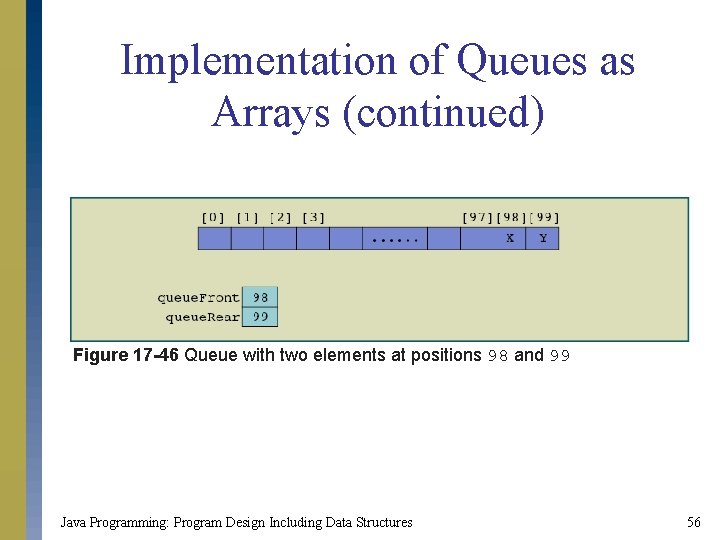Implementation of Queues as Arrays (continued) Figure 17 -46 Queue with two elements at positions 98 and 99 Java Programming: Program Design Including Data Structures 56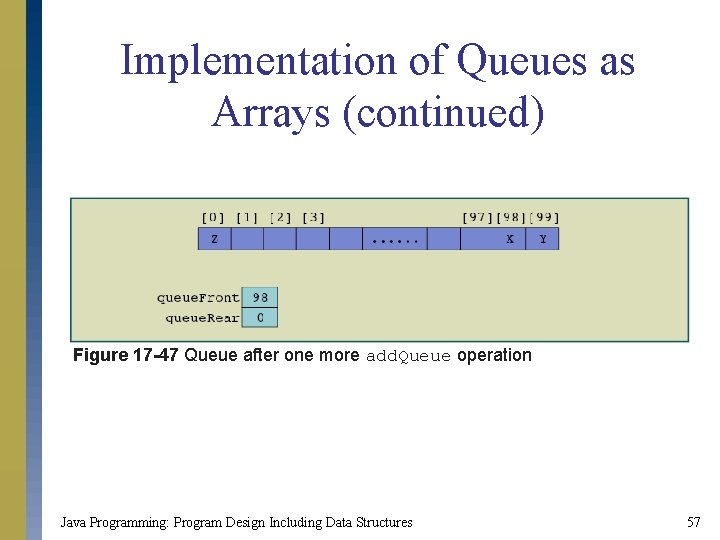Implementation of Queues as Arrays (continued) Figure 17 -47 Queue after one more add. Queue operation Java Programming: Program Design Including Data Structures 57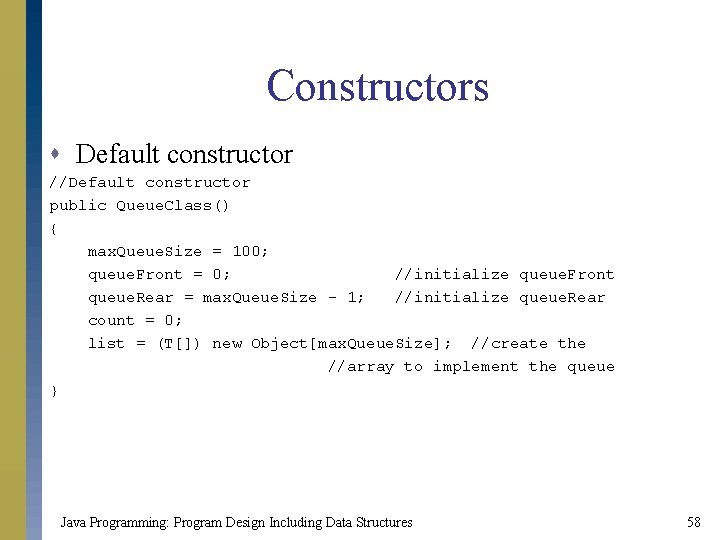Constructors s Default constructor //Default constructor public Queue. Class() { max. Queue. Size = 100; queue. Front = 0; //initialize queue. Front queue. Rear = max. Queue. Size - 1; //initialize queue. Rear count = 0; list = (T[]) new Object[max. Queue. Size]; //create the //array to implement the queue } Java Programming: Program Design Including Data Structures 58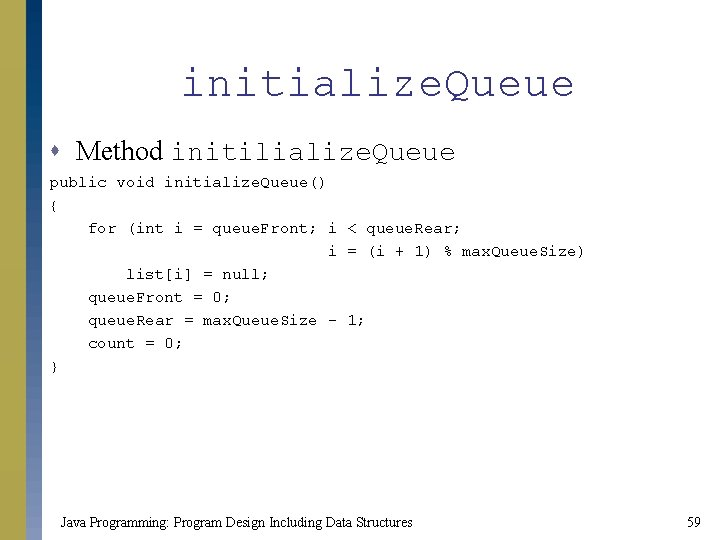initialize. Queue s Method initilialize. Queue public void initialize. Queue() { for (int i = queue. Front; i < queue. Rear; i = (i + 1) % max. Queue. Size) list[i] = null; queue. Front = 0; queue. Rear = max. Queue. Size - 1; count = 0; } Java Programming: Program Design Including Data Structures 59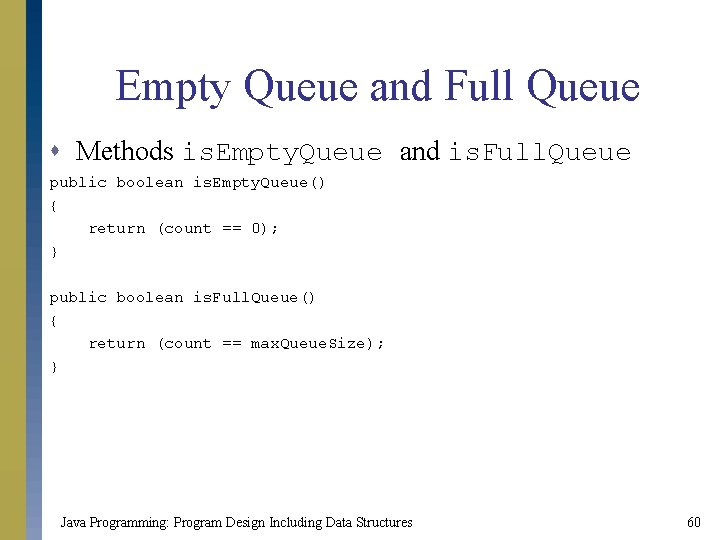Empty Queue and Full Queue s Methods is. Empty. Queue and is. Full. Queue public boolean is. Empty. Queue() { return (count == 0); } public boolean is. Full. Queue() { return (count == max. Queue. Size); } Java Programming: Program Design Including Data Structures 60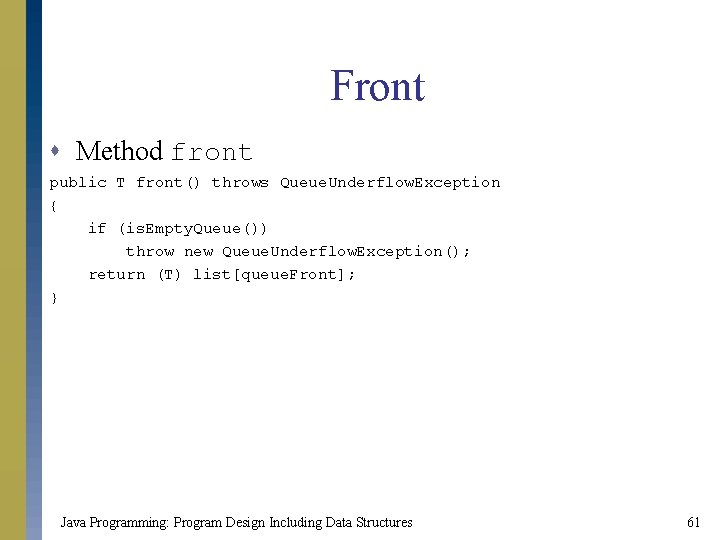Front s Method front public T front() throws Queue. Underflow. Exception { if (is. Empty. Queue()) throw new Queue. Underflow. Exception(); return (T) list[queue. Front]; } Java Programming: Program Design Including Data Structures 61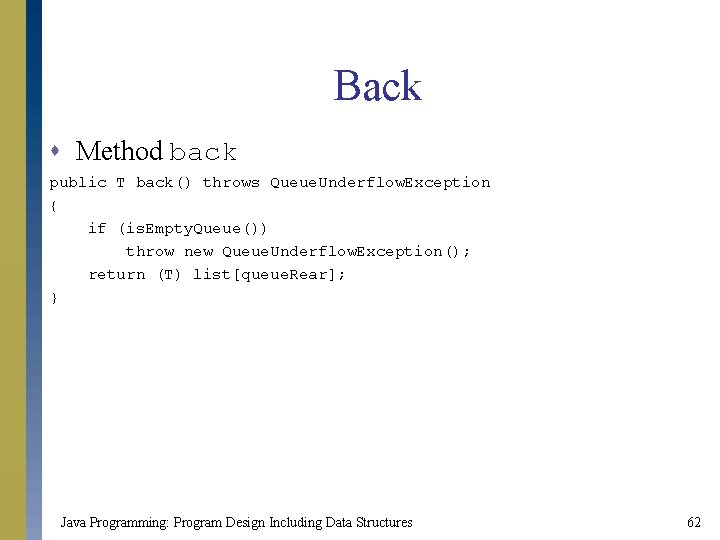Back s Method back public T back() throws Queue. Underflow. Exception { if (is. Empty. Queue()) throw new Queue. Underflow. Exception(); return (T) list[queue. Rear]; } Java Programming: Program Design Including Data Structures 62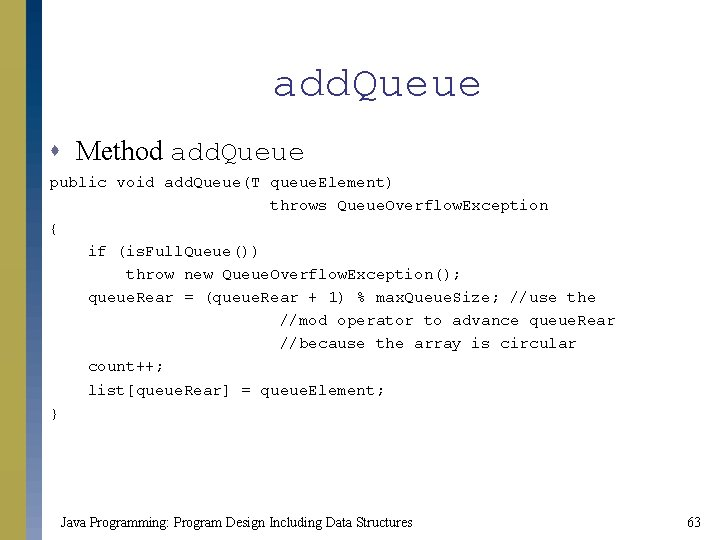add. Queue s Method add. Queue public void add. Queue(T queue. Element) throws Queue. Overflow. Exception { if (is. Full. Queue()) throw new Queue. Overflow. Exception(); queue. Rear = (queue. Rear + 1) % max. Queue. Size; //use the //mod operator to advance queue. Rear //because the array is circular count++; list[queue. Rear] = queue. Element; } Java Programming: Program Design Including Data Structures 63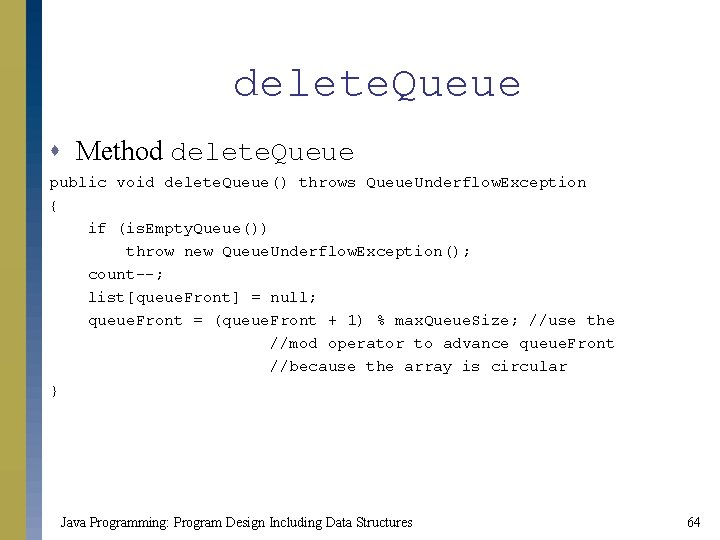delete. Queue s Method delete. Queue public void delete. Queue() throws Queue. Underflow. Exception { if (is. Empty. Queue()) throw new Queue. Underflow. Exception(); count--; list[queue. Front] = null; queue. Front = (queue. Front + 1) % max. Queue. Size; //use the //mod operator to advance queue. Front //because the array is circular } Java Programming: Program Design Including Data Structures 64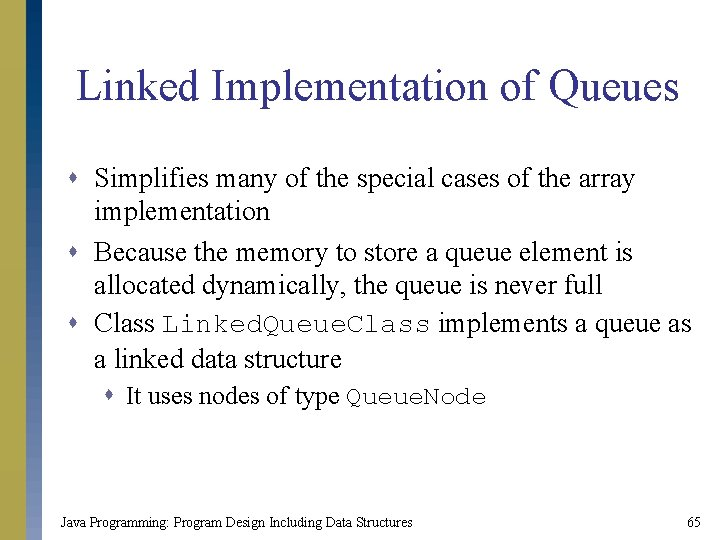Linked Implementation of Queues s Simplifies many of the special cases of the array implementation s Because the memory to store a queue element is allocated dynamically, the queue is never full s Class Linked. Queue. Class implements a queue as a linked data structure s It uses nodes of type Queue. Node Java Programming: Program Design Including Data Structures 65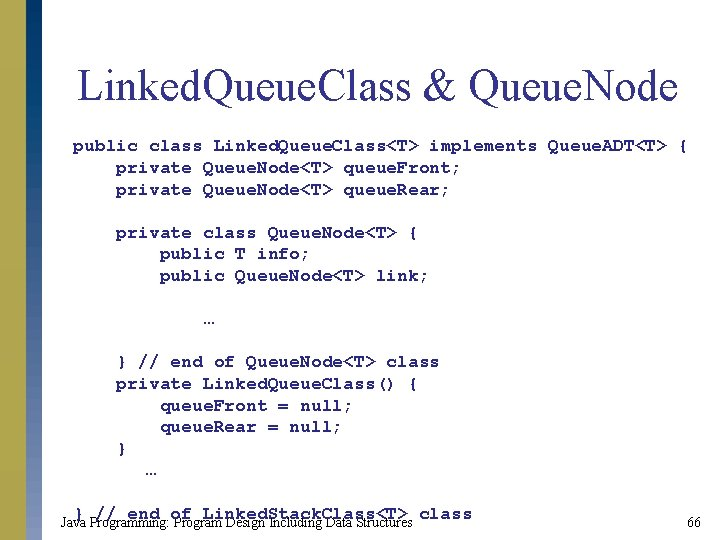Linked. Queue. Class & Queue. Node public class Linked. Queue. Class<T> implements Queue. ADT<T> { private Queue. Node<T> queue. Front; private Queue. Node<T> queue. Rear; private class Queue. Node<T> { public T info; public Queue. Node<T> link; … } // end of Queue. Node<T> class private Linked. Queue. Class() { queue. Front = null; queue. Rear = null; } … } // end of Linked. Stack. Class<T> class Java Programming: Program Design Including Data Structures 66Linked Implementation of Queues (continued) s Method initialize. Queue public void initialize. Queue() { queue. Front = null; queue. Rear = null; } Java Programming: Program Design Including Data Structures 67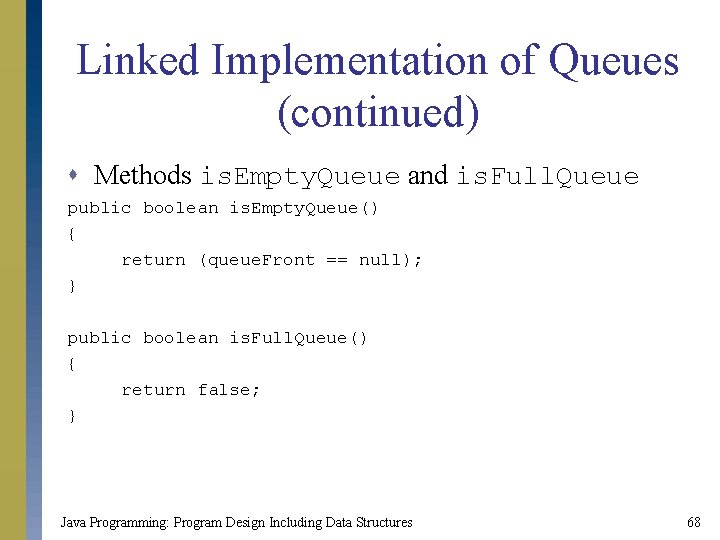Linked Implementation of Queues (continued) s Methods is. Empty. Queue and is. Full. Queue public boolean is. Empty. Queue() { return (queue. Front == null); } public boolean is. Full. Queue() { return false; } Java Programming: Program Design Including Data Structures 68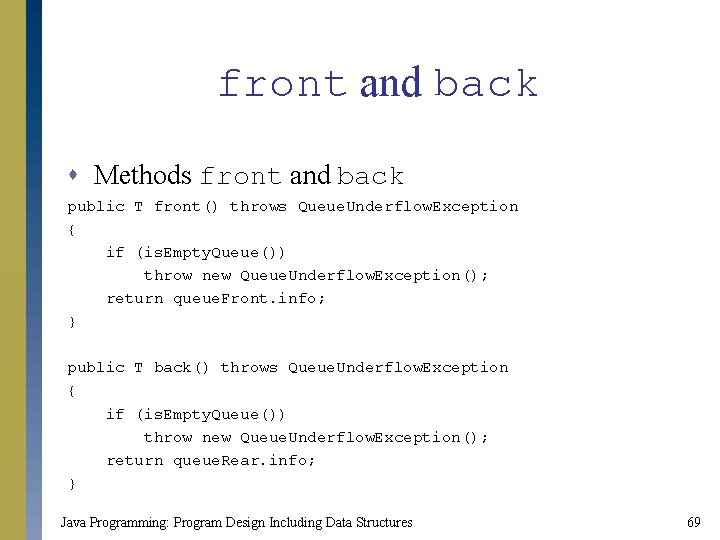front and back s Methods front and back public T front() throws Queue. Underflow. Exception { if (is. Empty. Queue()) throw new Queue. Underflow. Exception(); return queue. Front. info; } public T back() throws Queue. Underflow. Exception { if (is. Empty. Queue()) throw new Queue. Underflow. Exception(); return queue. Rear. info; } Java Programming: Program Design Including Data Structures 69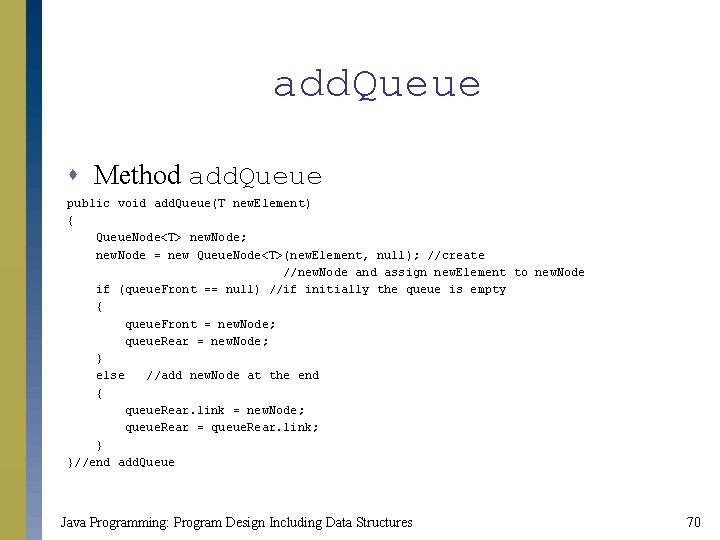add. Queue s Method add. Queue public void add. Queue(T new. Element) { Queue. Node<T> new. Node; new. Node = new Queue. Node<T>(new. Element, null); //create //new. Node and assign new. Element to new. Node if (queue. Front == null) //if initially the queue is empty { queue. Front = new. Node; queue. Rear = new. Node; } else //add new. Node at the end { queue. Rear. link = new. Node; queue. Rear = queue. Rear. link; } }//end add. Queue Java Programming: Program Design Including Data Structures 70delete. Queue s Method delete. Queue public void delete. Queue() throws Queue. Underflow. Exception { if (is. Empty. Queue()) throw new Queue. Underflow. Exception(); queue. Front = queue. Front. link; //advance queue. Front if (queue. Front == null) //if after deletion the queue. Rear = null; //is empty, set queue. Rear to null } //end delete. Queue Java Programming: Program Design Including Data Structures 71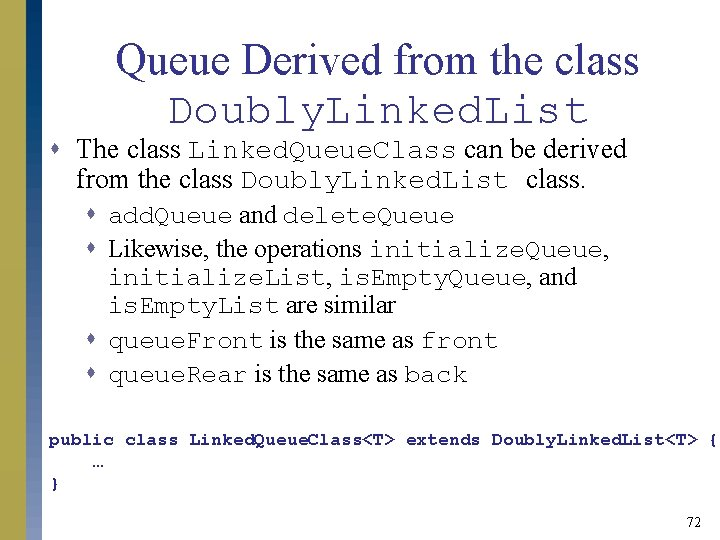Queue Derived from the class Doubly. Linked. List s The class Linked. Queue. Class can be derived from the class Doubly. Linked. List class. s add. Queue and delete. Queue s Likewise, the operations initialize. Queue, initialize. List, is. Empty. Queue, and is. Empty. List are similar s queue. Front is the same as front s queue. Rear is the same as back public class Linked. Queue. Class<T> extends Doubly. Linked. List<T> { … } 72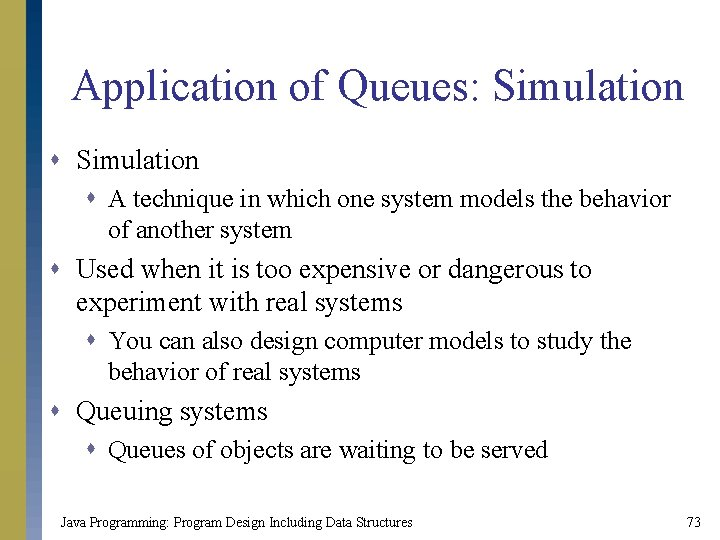Application of Queues: Simulation s A technique in which one system models the behavior of another system s Used when it is too expensive or dangerous to experiment with real systems s You can also design computer models to study the behavior of real systems s Queuing systems s Queues of objects are waiting to be served Java Programming: Program Design Including Data Structures 73Designing a Queuing System s Terms s Server s Object that provides a service s Customer s Object receiving a service s Transaction time s The time it takes to serve a customer Java Programming: Program Design Including Data Structures 74Designing a Queuing System (continued) s Components s List of servers s Queue of waiting objects s Process s The customer at the front of the queue waits for the next available server s When a server becomes free, the customer at the front of the queue moves to be served s At the beginning, all servers are free Java Programming: Program Design Including Data Structures 75Designing a Queuing System (continued) s What you need to know s s The number of servers The customer expected arrival time The time between the arrivals of customers The number of events affecting the system s Time-driven simulation s Uses a clock that can be implemented as a counter s The simulation is run for a fixed amount of time Java Programming: Program Design Including Data Structures 76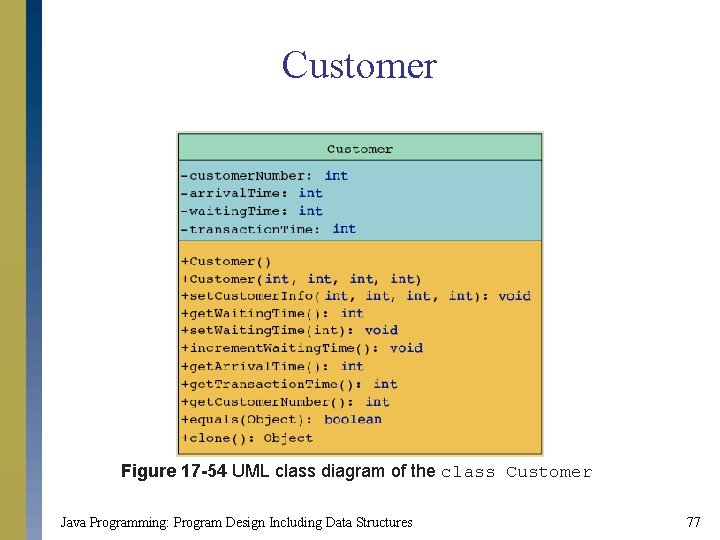Customer Figure 17 -54 UML class diagram of the class Customer Java Programming: Program Design Including Data Structures 77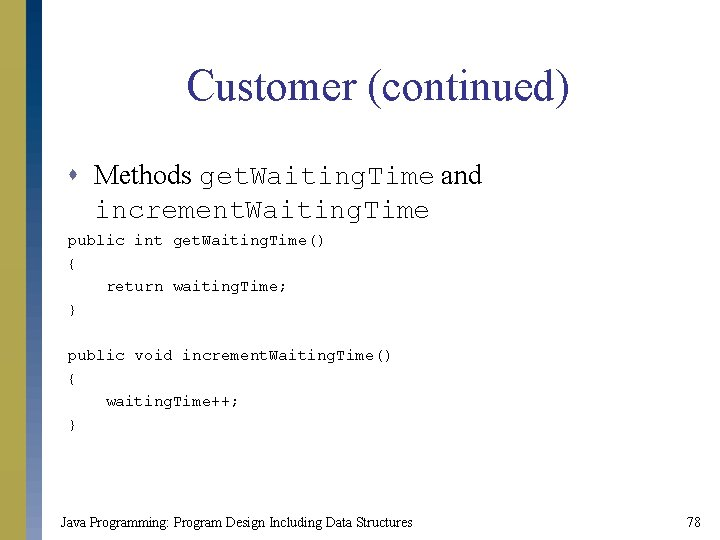Customer (continued) s Methods get. Waiting. Time and increment. Waiting. Time public int get. Waiting. Time() { return waiting. Time; } public void increment. Waiting. Time() { waiting. Time++; } Java Programming: Program Design Including Data Structures 78Server Figure 17 -55 UML class diagram of the class Server Java Programming: Program Design Including Data Structures 79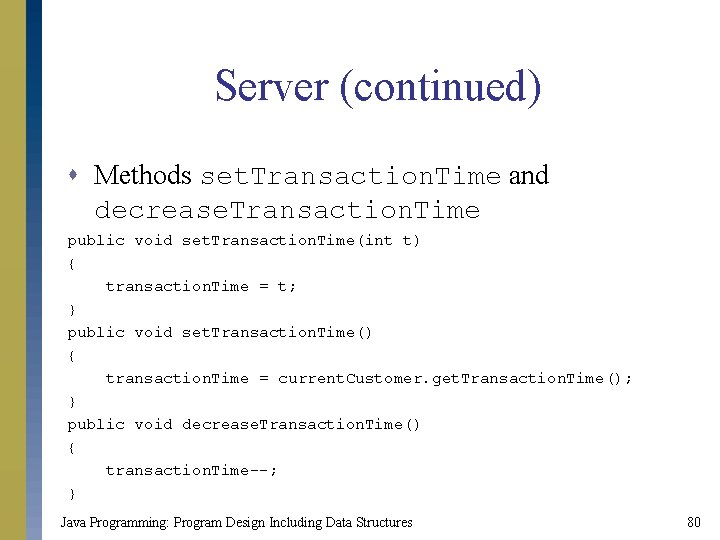Server (continued) s Methods set. Transaction. Time and decrease. Transaction. Time public void set. Transaction. Time(int t) { transaction. Time = t; } public void set. Transaction. Time() { transaction. Time = current. Customer. get. Transaction. Time(); } public void decrease. Transaction. Time() { transaction. Time--; } Java Programming: Program Design Including Data Structures 80Server List Figure 17 -56 UML class diagram of the class server. List Java Programming: Program Design Including Data Structures 81Server List (continued) s Method set. Server. Busy public void set. Server. Busy(int server. ID, Customer c. Customer, int t. Time) { servers[server. ID]. set. Busy(); servers[server. ID]. set. Transaction. Time(t. Time); servers[server. ID]. set. Current. Customer(c. Customer); } public void set. Server. Busy(int server. ID, Customer c. Customer) { int time; time = c. Customer. get. Transaction. Time(); servers[server. ID]. set. Busy(); servers[server. ID]. set. Transaction. Time(time); servers[server. ID]. set. Current. Customer(c. Customer); } Java Programming: Program Design Including Data Structures 82Waiting Customer Queue s Class Waiting. Customer. Queue class Waiting. Customer. Queue extends Queue. Class { public Waiting. Customer. Queue() { super(); } public Waiting. Customer. Queue(int size) { super(size); } public void update. Waiting. Queue() { //. . . } } Java Programming: Program Design Including Data Structures 83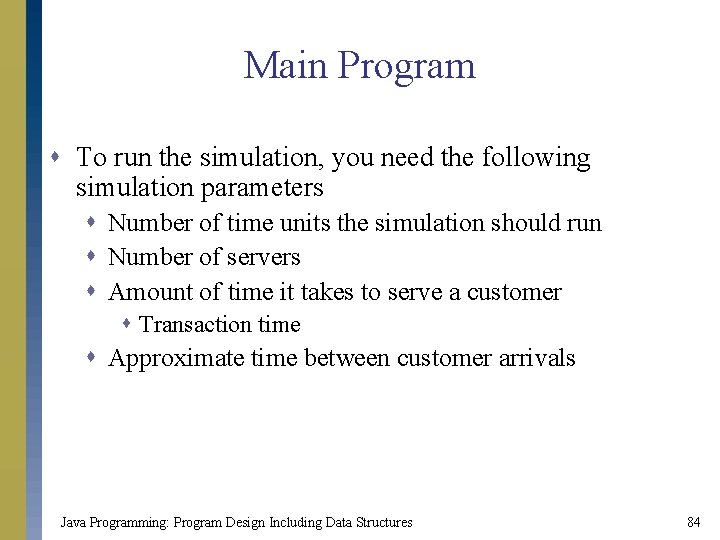Main Program s To run the simulation, you need the following simulation parameters s Number of time units the simulation should run s Number of servers s Amount of time it takes to serve a customer s Transaction time s Approximate time between customer arrivals Java Programming: Program Design Including Data Structures 84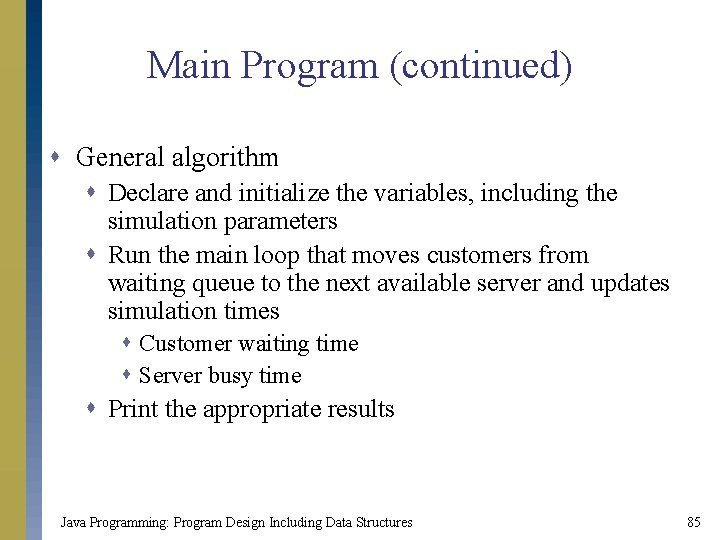Main Program (continued) s General algorithm s Declare and initialize the variables, including the simulation parameters s Run the main loop that moves customers from waiting queue to the next available server and updates simulation times s Customer waiting time s Server busy time s Print the appropriate results Java Programming: Program Design Including Data Structures 85Chapter Summary s Stacks s Addition and deletion of elements occur only at one end, called the top of the stack s Follow a LIFO policy s Stack implementation s Array-based stacks s Linked stacks s Stacks are used to convert recursive algorithms into nonrecursive algorithms Java Programming: Program Design Including Data Structures 86Chapter Summary (continued) s Queues s Elements are added at one end, called the rear, and deleted from the other end, called the front s Follow a FIFO policy s Queue implementation s Array-based queues s Linked queues s Queues are used to construct computer-aided simulation programs Java Programming: Program Design Including Data Structures 87